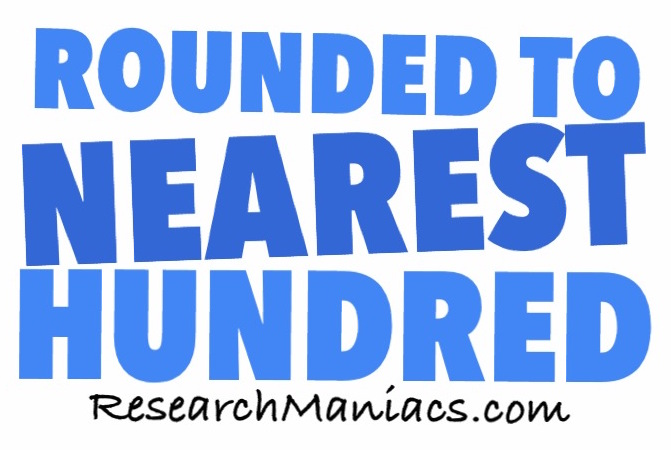What is 452 rounded to the nearest hundred?Here we will tell you what 452 is rounded to the nearest hundred and also show you what rules we used to get to the answer.

Remember, we did not necessarily round up or down, but to the hundred that is nearest to 452.

First, 452 rounded to the nearest hundred is:

500

When rounding to the nearest hundred, like we did with 452 above, we use the following rules:

A) We round the number up to the nearest hundred if the last two digits in the number are 50 or above.

B) We round the number down to the nearest hundred if the last two digits in the number are 49 or below.

C) If the last two digits are 00, then we do not have to do any rounding, because it is already to the hundred.

Rounded to Nearest Hundred
Enter another number below to round it to the nearest hundred.

What is 453 rounded to the nearest hundred?
Now you know what 452 rounded to the nearest hundred is. Go here for the next number we rounded.